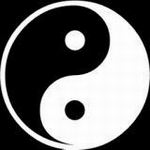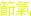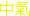The Chinese CalendarFor example, the Gregorian calendar is a solar calendar with a year length of 365 days. It has one leap day every four years (29 February), except when the year is divisible by 100 but not by 400. In a period of 400 years there will be 100 - 4 + 1 leap years, therefore the number of days in a 400 year period equals 400*365 +97 = 146097, a difference of less than 3 hours (!) with the actual number of days 400*365.24219=146096.876 It will be clear that in this calendar there is no connection with the lunar cycle.
 As another example the Islamic calendar has years which consist of 12 months with alternatively 30 and 29 days. If this would be all, there would remain in one year a difference of 12*(29.5306-29.5)=0.3672 day (about 9 hours). In 30 years this difference would grow to 11.016 days. Therefore in a 30 year period 11 leap days have to be added. The last month of the year will in a 30 year period have 19 times a length of 29 days and 11 times an extra 30th day. As a result there will remain a difference of 0.016 days in a period of 30 years Obviously in this calendar there is no connection with the solar cycle, the Islamic year is roughly 11 days shorter than the solar year.The Chinese calendar is an example of a lunisolar calendar, which tries to combine lunar months with a solar year. In the Chinese calendar, months are based on the lunar cycle: the first day of a month is the day in which the new moon occurs. This means that the length of a month will be either 29 or 30 days and that the length of a specific month will not be the same every year. As 12 months make a lunar year of 354.3672 days, there is the same difference of roughly 11 days with the solar year as in the Islamic calendar. To keep the calendar synchronised with the solar year an extra leap month is added on the average every 3 years. Because of this the length of a year in the Chinese calendar can vary considerably. Careful analysis shows that it can have 353, 354 or 355 days in a normal year and 383, 384 or 385 days in a leap year. When will there be a leap month? To understand this we must first introduce the concept of solar markers.The Winter and Summer solstices and the Vernal and Autumnal equinoxes can be called solor markers which divide the ecliptic in 4 sectors of 90 o . The 24 Chinese solar markers do the same, but in sectors of 15 degrees each. The first marker coincides with a position of the sun at a longitude of 315o. This is the middle between the Winter solstice (315o) and the Vernal equinox (360o), around the 4th of Februari and is called the Beginning of Spring. The second one falls on the 19th of February and is called Rain Water. And so on. The odd numbered ones are the Setoff Points ()of what can be called solar months and the even numbered ones are the Center Points () of these months. For example: the first solar month (called the Tiger month), starts at the set off point of the Beginning of spring and has at its Center point Rain Water. The next solar month (Rabbit month) would then start around the 6th of March and would have its Center Point at the Vernal equinox on the 21th of March. In the same way the Summer Solstice is the Center Point of the 5th month (Horse) and the Autumnal equinox is the Center point of the 8th month (Chicken) Note that in the Chinese calendar Spring starts NOT on the vernal equinox, but half way between Winter solstice and Vernal equinox. The same holds for the other seasons. If the motion of the earth around the sun would be uniform, the length of any solar month would be 365.24219/12 = 30.43685 days, and also the time between consecutive Center points would be the same number of days. But then it can happen that a lunar month (29.5306 days) doesn't contain a Center Point! This gives us the 'simple' rule for leap months:When a lunar month doesn't contain a Solar Center Point, it is a leap month and it will have the same name as the preceding month.As a result, the year in which this occurs will have 13 months.